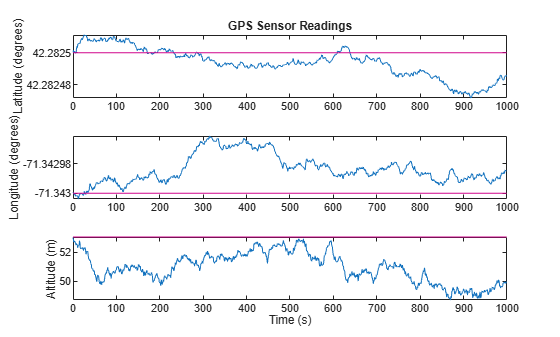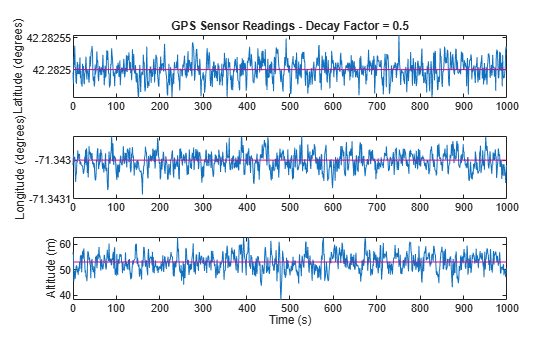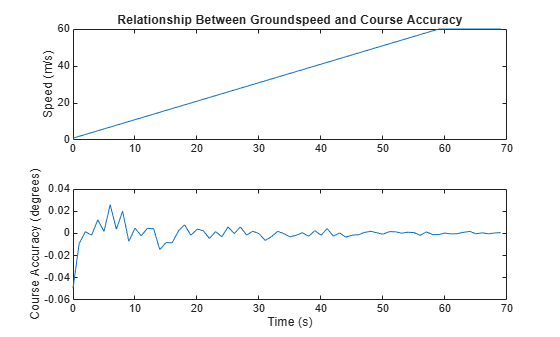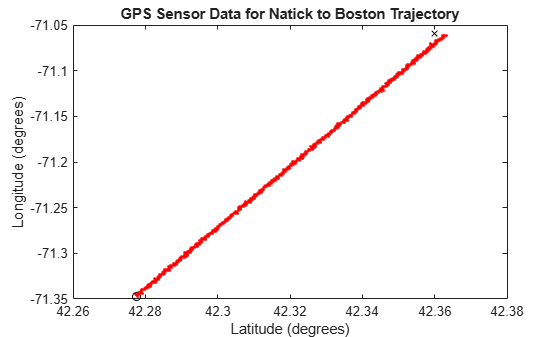# gpsSensor

## Description

The `gpsSensor` System object™ models data output from a Global Positioning System (GPS) receiver.

1. Create the `gpsSensor` object and set its properties.

2. Call the object with arguments, as if it were a function.

## Creation

### Syntax

``GPS = gpsSensor``
``GPS = gpsSensor('ReferenceFrame',RF)``
``GPS = gpsSensor(___,Name,Value)``

### Description

````GPS = gpsSensor` returns a `gpsSensor` System object that computes a Global Positioning System receiver reading based on a local position and velocity input signal. The default reference position in geodetic coordinates is latitude: 0o Nlongitude: 0o Ealtitude: 0 m ```
````GPS = gpsSensor('ReferenceFrame',RF)` returns a `gpsSensor` System object that computes a global positioning system receiver reading relative to the reference frame `RF`. Specify `RF` as `'NED'` (North-East-Down) or `'ENU'`(East-North-Up). The default value is `'NED'`. ```

example

````GPS = gpsSensor(___,Name,Value)` sets each property `Name` to the specified `Value`. Unspecified properties have default values.```

## Properties

expand all

Unless otherwise indicated, properties are nontunable, which means you cannot change their values after calling the object. Objects lock when you call them, and the `release` function unlocks them.

If a property is tunable, you can change its value at any time.

Update rate of the receiver in Hz, specified as a positive real scalar.

Data Types: `single` | `double`

Reference location, specified as a 3-element row vector in geodetic coordinates (latitude, longitude, and altitude). Altitude is the height above the reference ellipsoid model, WGS84. The reference location is in [degrees degrees meters]. The degree format is decimal degrees (DD).

Data Types: `single` | `double`

Position coordinate input format, specified as `'Local'` or `'Geodetic'`.

• If you set the property as `'Local'`, then you need to specify the `truePosition` input as Cartesian coordinates with respect to the local navigation frame whose origin is fixed and defined by the `ReferenceLcation` property. Additionally, when you specify the `trueVelocity` input, you need to specify it with respect to this local navigation frame.

• If you set the property as `'Geodetic'`, then you need to specify the `truePosition` input as geodetic coordinates in latitude, longitude, and altitude. Additionally, when you specify the `trueVelocity` input, you need to specify it with respect to the navigation frame (NED or ENU) whose origin corresponds to the `truePosition` input. When setting the property as `'Geodetic'`, the `gpsSensor` object neglects the `ReferenceLocation` property.

Data Types: `character vector`

Horizontal position accuracy in meters, specified as a nonnegative real scalar. The horizontal position accuracy specifies the standard deviation of the noise in the horizontal position measurement.

Tunable: Yes

Data Types: `single` | `double`

Vertical position accuracy in meters, specified as a nonnegative real scalar. The vertical position accuracy specifies the standard deviation of the noise in the vertical position measurement.

Tunable: Yes

Data Types: `single` | `double`

Velocity accuracy in meters per second, specified as a nonnegative real scalar. The velocity accuracy specifies the standard deviation of the noise in the velocity measurement.

Tunable: Yes

Data Types: `single` | `double`

Global position noise decay factor, specified as a scalar in the range [0,1].

A decay factor of 0 models the global position noise as a white noise process. A decay factor of 1 models the global position noise as a random walk process.

Tunable: Yes

Data Types: `single` | `double`

Random number source, specified as a character vector or string:

• `'Global stream'` –– Random numbers are generated using the current global random number stream.

• `'mt19937ar with seed'` –– Random numbers are generated using the mt19937ar algorithm with the seed specified by the `Seed` property.

Data Types: `char` | `string`

Initial seed of an mt19937ar random number generator algorithm, specified as a nonnegative integer scalar.

#### Dependencies

To enable this property, set `RandomStream` to `'mt19937ar with seed'`.

Data Types: `single` | `double` | `int8` | `int16` | `int32` | `int64` | `uint8` | `uint16` | `uint32` | `uint64`

## Usage

### Syntax

``[position,velocity,groundspeed,course] = GPS(truePosition,trueVelocity)``

### Description

example

````[position,velocity,groundspeed,course] = GPS(truePosition,trueVelocity)` computes global navigation satellite system receiver readings from the position and velocity inputs.```

### Input Arguments

expand all

Position of the GPS receiver in the navigation coordinate system, specified as a real finite N-by-3 matrix. N is the number of samples in the current frame.

• When the `PositionInputFormat` property is specified as `'Local'`, specify `turePosition` as Cartesian coordinates with respect to the local navigation frame whose origin is fixed at `ReferenceLocation`.

• When the `PositionInputFormat` property is specified as `'Geodetic'`, specify `turePosition` as geodetic coordinates in `[latitude,longitude,altitude]`. `Latitude` and `longitude` are in meters. `altitude` is the height above the WGS84 ellipsoid model in meters.

Data Types: `single` | `double`

Velocity of GPS receiver in the navigation coordinate system in meters per second, specified as a real finite N-by-3 matrix. N is the number of samples in the current frame.

• When the `PositionInputFormat` property is specified as `'Local'`, specify `trueVelocity` with respect to the local navigation frame (NED or ENU) whose origin is fixed at `ReferenceLocation`.

• When the `PositionInputFormat` property is specified as `'Geodetic'`, specify `trueVelocity` with respect to the navigation frame (NED or ENU) whose origin corresponds to the `truePosition` input.

Data Types: `single` | `double`

### Output Arguments

expand all

Position of the GPS receiver in the geodetic latitude, longitude, and altitude (LLA) coordinate system, returned as a real finite N-by-3 array. Latitude and longitude are in degrees with North and East being positive. Altitude is in meters.

N is the number of samples in the current frame.

Data Types: `single` | `double`

Velocity of the GPS receiver in the local navigation coordinate system in meters per second, returned as a real finite N-by-3 array. N is the number of samples in the current frame.

• When the `PositionInputFormat` property is specified as `'Local'`, the returned velocity is with respect to the local navigation frame whose origin is fixed at `ReferenceLocation`.

• When the `PositionInputFormat` property is specified as `'Geodetic'`, the returned velocity is with respect to the navigation frame (NED or ENU) whose origin corresponds to the `position` output.

Data Types: `single` | `double`

Magnitude of the horizontal velocity of the GPS receiver in the local navigation coordinate system in meters per second, returned as a real finite N-by-1 column vector.

N is the number of samples in the current frame.

Data Types: `single` | `double`

Direction of the horizontal velocity of the GPS receiver in the local navigation coordinate system in degrees, returned as a real finite N-by-1 column of values between 0 and 360. North corresponds to 360 degrees and East corresponds to 90 degrees.

N is the number of samples in the current frame.

Data Types: `single` | `double`

## Object Functions

To use an object function, specify the System object as the first input argument. For example, to release system resources of a System object named `obj`, use this syntax:

`release(obj)`

expand all

 `step` Run System object algorithm `release` Release resources and allow changes to System object property values and input characteristics `reset` Reset internal states of System object

## Examples

collapse all

Create a `gpsSensor` System object™ to model GPS receiver data. Assume a typical one Hz sample rate and a 1000-second simulation time. Define the reference location in terms of latitude, longitude, and altitude (LLA) of Natick, MA (USA). Define the sensor as stationary by specifying the true position and velocity with zeros.

```fs = 1; duration = 1000; numSamples = duration*fs; refLoc = [42.2825 -71.343 53.0352]; truePosition = zeros(numSamples,3); trueVelocity = zeros(numSamples,3); gps = gpsSensor('SampleRate',fs,'ReferenceLocation',refLoc);```

Call `gps` with the specified `truePosition` and `trueVelocity` to simulate receiving GPS data for a stationary platform.

`position = gps(truePosition,trueVelocity);`

Plot the true position and the GPS sensor readings for position.

```t = (0:(numSamples-1))/fs; subplot(3, 1, 1) plot(t, position(:,1), ... t, ones(numSamples)*refLoc(1)) title('GPS Sensor Readings') ylabel('Latitude (degrees)') subplot(3, 1, 2) plot(t, position(:,2), ... t, ones(numSamples)*refLoc(2)) ylabel('Longitude (degrees)') subplot(3, 1, 3) plot(t, position(:,3), ... t, ones(numSamples)*refLoc(3)) ylabel('Altitude (m)') xlabel('Time (s)')```The position readings have noise controlled by `HorizontalPositionAccuracy`, `VerticalPositionAccuracy`, `VelocityAccuracy`, and `DecayFactor`. The `DecayFactor` property controls the drift in the noise model. By default, `DecayFactor` is set to `0.999`, which approaches a random walk process. To observe the effect of the `DecayFactor` property:

1. Reset the `gps` object.

2. Set `DecayFactor` to `0.5`.

3. Call `gps` with variables specifying a stationary position.

4. Plot the results.

The `GPS` position readings now oscillate around the true position.

```reset(gps) gps.DecayFactor = 0.5; position = gps(truePosition,trueVelocity); subplot(3, 1, 1) plot(t, position(:,1), ... t, ones(numSamples)*refLoc(1)) title('GPS Sensor Readings - Decay Factor = 0.5') ylabel('Latitude (degrees)') subplot(3, 1, 2) plot(t, position(:,2), ... t, ones(numSamples)*refLoc(2)) ylabel('Longitude (degrees)') subplot(3, 1, 3) plot(t, position(:,3), ... t, ones(numSamples)*refLoc(3)) ylabel('Altitude (m)') xlabel('Time (s)')```GPS receivers achieve greater course accuracy as groundspeed increases. In this example, you create a GPS receiver simulation object and simulate the data received from a platform that is accelerating from a stationary position.

Create a default `gpsSensor` System object™ to model data returned by a GPS receiver.

`GPS = gpsSensor`
```GPS = gpsSensor with properties: SampleRate: 1 Hz PositionInputFormat: 'Local' ReferenceLocation: [0 0 0] [deg deg m] HorizontalPositionAccuracy: 1.6 m VerticalPositionAccuracy: 3 m VelocityAccuracy: 0.1 m/s RandomStream: 'Global stream' DecayFactor: 0.999 ```

Create matrices to describe the position and velocity of a platform in the NED coordinate system. The platform begins from a stationary position and accelerates to 60 m/s North-East over 60 seconds, then has a vertical acceleration to 2 m/s over 2 seconds, followed by a 2 m/s rate of climb for another 8 seconds. Assume a constant velocity, such that the velocity is the simple derivative of the position.

```duration = 70; numSamples = duration*GPS.SampleRate; course = 45*ones(duration,1); groundspeed = [(1:60)';60*ones(10,1)]; Nvelocity = groundspeed.*sind(course); Evelocity = groundspeed.*cosd(course); Dvelocity = [zeros(60,1);-1;-2*ones(9,1)]; NEDvelocity = [Nvelocity,Evelocity,Dvelocity]; Ndistance = cumsum(Nvelocity); Edistance = cumsum(Evelocity); Ddistance = cumsum(Dvelocity); NEDposition = [Ndistance,Edistance,Ddistance];```

Model GPS measurement data by calling the `GPS` object with your velocity and position matrices.

`[~,~,groundspeedMeasurement,courseMeasurement] = GPS(NEDposition,NEDvelocity);`

Plot the groundspeed and the difference between the true course and the course returned by the GPS simulator.

As groundspeed increases, the accuracy of the course increases. Note that the velocity increase during the last ten seconds has no effect, because the additional velocity is not in the ground plane.

```t = (0:numSamples-1)/GPS.SampleRate; subplot(2,1,1) plot(t,groundspeed); ylabel('Speed (m/s)') title('Relationship Between Groundspeed and Course Accuracy') subplot(2,1,2) courseAccuracy = courseMeasurement - course; plot(t,courseAccuracy) xlabel('Time (s)'); ylabel('Course Accuracy (degrees)')```Simulate GPS data received during a trajectory from the city of Natick, MA, to Boston, MA.

Define the decimal degree latitude and longitude for the city of Natick, MA USA, and Boston, MA USA. For simplicity, set the altitude for both locations to zero.

```NatickLLA = [42.27752809999999, -71.34680909999997, 0]; BostonLLA = [42.3600825, -71.05888010000001, 0];```

Define a motion that can take a platform from Natick to Boston in 20 minutes. Set the origin of the local NED coordinate system as Natick. Create a `waypointTrajectory` object to output the trajectory 10 samples at a time.

```fs = 1; duration = 60*20; bearing = 68; % degrees distance = 25.39e3; % meters distanceEast = distance*sind(bearing); distanceNorth = distance*cosd(bearing); NatickNED = [0,0,0]; BostonNED = [distanceNorth,distanceEast,0]; trajectory = waypointTrajectory( ... 'Waypoints', [NatickNED;BostonNED], ... 'TimeOfArrival',[0;duration], ... 'SamplesPerFrame',10, ... 'SampleRate',fs);```

Create a `gpsSensor` object to model receiving GPS data for the platform. Set the `HorizontalPositionalAccuracy` to `25` and the `DecayFactor` to `0.25` to emphasize the noise. Set the `ReferenceLocation` to the Natick coordinates in LLA.

```GPS = gpsSensor( ... 'HorizontalPositionAccuracy',25, ... 'DecayFactor',0.25, ... 'SampleRate',fs, ... 'ReferenceLocation',NatickLLA);```

Open a figure and plot the position of Natick and Boston in LLA. Ignore altitude for simplicity.

In a loop, call the `gpsSensor` object with the ground-truth trajectory to simulate the received GPS data. Plot the ground-truth trajectory and the model of received GPS data.

```figure(1) plot(NatickLLA(1),NatickLLA(2),'ko', ... BostonLLA(1),BostonLLA(2),'kx') xlabel('Latitude (degrees)') ylabel('Longitude (degrees)') title('GPS Sensor Data for Natick to Boston Trajectory') hold on while ~isDone(trajectory) [truePositionNED,~,trueVelocityNED] = trajectory(); reportedPositionLLA = GPS(truePositionNED,trueVelocityNED); figure(1) plot(reportedPositionLLA(:,1),reportedPositionLLA(:,2),'r.') end```As a best practice, release System objects when complete.

```release(GPS) release(trajectory)```

## Extended Capabilities

### Objects

Introduced in R2020b

## SupportGet trial now